1594957980

# What is k-Nearest Neighbor?

It is one of the simplest supervise learning techniques. If there is a new data point that we need to classify then we will choose k “closest” data points (nearest neighbors) that will be around the new data point for performing classification or regression. K can be any positive number.

KNN is a Non-parametric algorithm i.e. it does not make any underlying assumptions about the distribution of data. It is used for both classifications and regression problems.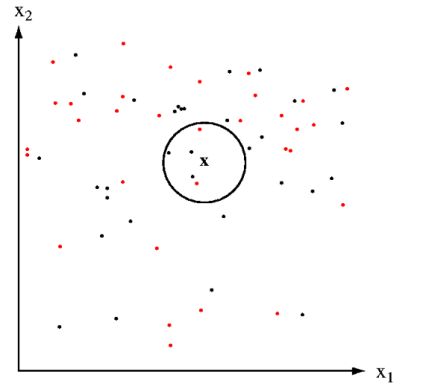Example: We have two categorical variables red and black, and we have to classify a new data point X whether it belongs to red or black.

We select K=5 and select five close data points near to X. As you can see in the above figure in 5 data point 3 is black and 2 is red since black in a majority in number the new data point X will belong to a black class.

**KNN for Regression: **When KNN is used for regression problems the prediction is based on the mean or the median of the nearest neighbor.

**KNN for Classification: **Inclassification, the output can be calculated as the class with the highest occurrence from the K nearest neighbor.

# How does the KNN algorithm works?

The KNN algorithm work with the below steps:

• Step-1: Select the number K of the neighbors
• Step-2: Calculate the distance of K number of neighbors
• Step-3: Select the K nearest neighbors as per the calculated distance.
• Step-4: Among these k neighbors, count the number of the data points in each category.
• Step-5: Assign the new data points to that category for which have the highest number.

Suppose we have a new data point and we need to put the new data point in the correct category. Consider the below image: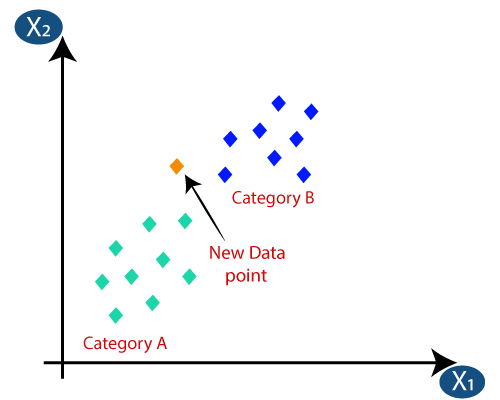• First, we need to choose the K number of neighbors, so we will choose the k=5.
• Next, we will calculate the distance between the data points.

We can any of the three functions to calculate the distance between the data point to find the closest data point. In this example, we will use the Euclidean distance.

#knn #data-science #knn-algorithm #algorithms

## Buddha Community1593571140

## K-Nearest Neighbors

A perfect opening line I must say for presenting the K-Nearest Neighbors. Yes, that’s how simple the concept behind KNN is. It just classifies a data point based on its few nearest neighbors. How many neighbors? That is what we decide.

Looks like you already know a lot of there is to know about this simple model. Let’s dive in to have a much closer look.

Before moving on, it’s important to know that KNN can be used for both classification and regression problems. We will first understand how it works for a classification problem, thereby making it easier to visualize regression.

## KNN Classifier

The data we are going to use is the Breast Cancer Wisconsin(Diagnostic) Data Set_. _There are 30 attributes that correspond to the real-valued features computed for a cell nucleus under consideration. A total of 569 such samples are present in this data, out of which 357 are classified as ‘benign’ (harmless) and the rest 212 are classified as _‘malignant’ _(harmful).

The diagnosis column contains ‘M’ or ‘B’ values for malignant and benign cancers respectively. I have changed these values to 1 and 0 respectively, for better analysis.

Also, for the sake of this post, I will only use two attributes from the data → ‘mean radius’ and ‘mean texture’. This will later help us visualize the decision boundaries drawn by KNN. Here’s how the final data looks like (after shuffling):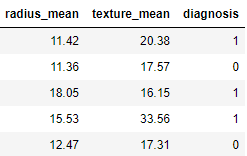Let’s code the KNN:

``````# Defining X and y
X = data.drop('diagnosis',axis=1)
y = data.diagnosis

# Splitting data into train and test
from sklearn.model_selection import train_test_split
X_train, X_test, y_train, y_test = train_test_split(X,y,test_size=0.25,random_state=42)
# Importing and fitting KNN classifier for k=3
from sklearn.neighbors import KNeighborsClassifier
knn = KNeighborsClassifier(n_neighbors=3)
knn.fit(X_train,y_train)
# Predicting results using Test data set
pred = knn.predict(X_test)
from sklearn.metrics import accuracy_score
accuracy_score(pred,y_test)
``````

The above code should give you the following output with a slight variation.

``````0.8601398601398601
``````

What just happened? When we trained the KNN on training data, it took the following steps for each data sample:

1. Calculate the distance between the data sample and every other sample with the help of a method such as Euclidean.
2. Sort these values of distances in ascending order.
3. Choose the top K values from the sorted distances.
4. Assign the class to the sample based on the most frequent class in the above K values.

Let’s visualize how KNN drew a decision boundary on the train data set and how the same boundary is then used to classify the test data set.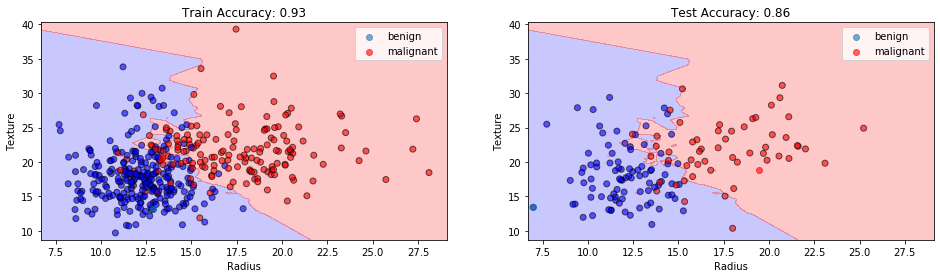KNN Classification at K=3. Image by Sangeet Aggarwal

With the training accuracy of 93% and the test accuracy of 86%, our model might have shown overfitting here. Why so?

When the value of K or the number of neighbors is too low, the model picks only the values that are closest to the data sample, thus forming a very complex decision boundary as shown above. Such a model fails to generalize well on the test data set, thereby showing poor results.

The problem can be solved by tuning the value of _n_neighbors _parameter. As we increase the number of neighbors, the model starts to generalize well, but increasing the value too much would again drop the performance.

Therefore, it’s important to find an optimal value of K, such that the model is able to classify well on the test data set. Let’s observe the train and test accuracies as we increase the number of neighbors.

#knn-algorithm #data-science #knn #nearest-neighbors #machine-learning #algorithms1594957980

# What is k-Nearest Neighbor?

It is one of the simplest supervise learning techniques. If there is a new data point that we need to classify then we will choose k “closest” data points (nearest neighbors) that will be around the new data point for performing classification or regression. K can be any positive number.

KNN is a Non-parametric algorithm i.e. it does not make any underlying assumptions about the distribution of data. It is used for both classifications and regression problems.Example: We have two categorical variables red and black, and we have to classify a new data point X whether it belongs to red or black.

We select K=5 and select five close data points near to X. As you can see in the above figure in 5 data point 3 is black and 2 is red since black in a majority in number the new data point X will belong to a black class.

**KNN for Regression: **When KNN is used for regression problems the prediction is based on the mean or the median of the nearest neighbor.

**KNN for Classification: **Inclassification, the output can be calculated as the class with the highest occurrence from the K nearest neighbor.

# How does the KNN algorithm works?

The KNN algorithm work with the below steps:

• Step-1: Select the number K of the neighbors
• Step-2: Calculate the distance of K number of neighbors
• Step-3: Select the K nearest neighbors as per the calculated distance.
• Step-4: Among these k neighbors, count the number of the data points in each category.
• Step-5: Assign the new data points to that category for which have the highest number.

Suppose we have a new data point and we need to put the new data point in the correct category. Consider the below image:• First, we need to choose the K number of neighbors, so we will choose the k=5.
• Next, we will calculate the distance between the data points.

We can any of the three functions to calculate the distance between the data point to find the closest data point. In this example, we will use the Euclidean distance.

#knn #data-science #knn-algorithm #algorithms1597235100

## k nearest neighbors computational complexity

### Understanding the computational cost of kNN algorithm, with case study examples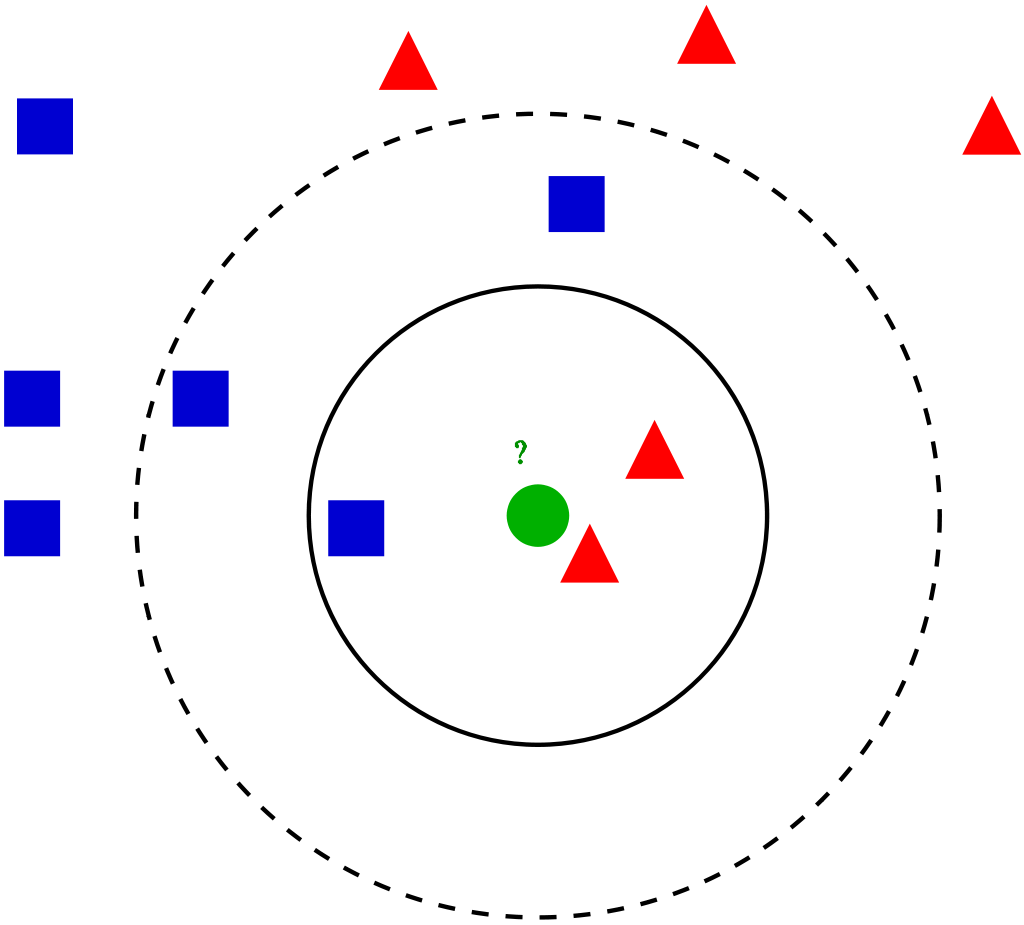Visualization of the kNN algorithm (source)

## Algorithm introduction

kNN (k nearest neighbors) is one of the simplest ML algorithms, often taught as one of the first algorithms during introductory courses. It’s relatively simple but quite powerful, although rarely time is spent on understanding its computational complexity and practical issues. It can be used both for classification and regression with the same complexity, so for simplicity, we’ll consider the kNN classifier.

kNN is an associative algorithm — during prediction it searches for the nearest neighbors and takes their majority vote as the class predicted for the sample. Training phase may or may not exist at all, as in general, we have 2 possibilities:

1. Brute force method — calculate distance from new point to every point in training data matrix X, sort distances and take k nearest, then do a majority vote. There is no need for separate training, so we only consider prediction complexity.
2. Using data structure — organize the training points from X into the auxiliary data structure for faster nearest neighbors lookup. This approach uses additional space and time (for creating data structure during training phase) for faster predictions.

We focus on the methods implemented in Scikit-learn, the most popular ML library for Python. It supports brute force, k-d tree and ball tree data structures. These are relatively simple, efficient and perfectly suited for the kNN algorithm. Construction of these trees stems from computational geometry, not from machine learning, and does not concern us that much, so I’ll cover it in less detail, more on the conceptual level. For more details on that, see links at the end of the article.

In all complexities below times of calculating the distance were omitted since they are in most cases negligible compared to the rest of the algorithm. Additionally, we mark:

• `n`: number of points in the training dataset
• `d`: data dimensionality
• `k`: number of neighbors that we consider for voting

## Brute force method

Training time complexity: `O(1)`

**Training space complexity: **`O(1)`

Prediction time complexity: `O(k * n)`

Prediction space complexity: `O(1)`

Training phase technically does not exist, since all computation is done during prediction, so we have `O(1)` for both time and space.

Prediction phase is, as method name suggest, a simple exhaustive search, which in pseudocode is:

``````Loop through all points k times:
1\. Compute the distance between currently classifier sample and
training points, remember the index of the element with the
smallest distance (ignore previously selected points)
2\. Add the class at found index to the counter
Return the class with the most votes as a prediction
``````

This is a nested loop structure, where the outer loop takes `k` steps and the inner loop takes `n` steps. 3rd point is `O(1)` and 4th is `O(## of classes)`, so they are smaller. Therefore, we have `O(n * k)` time complexity.

As for space complexity, we need a small vector to count the votes for each class. It’s almost always very small and is fixed, so we can treat is as a `O(1)` space complexity.

#k-nearest-neighbours #knn-algorithm #knn #machine-learning #algorithms1602838800

## What is kNN?

k-Nearest Neighbors is one of the easiest Machine Learning algorithms. It is a “Classification” algorithm to be specific. But due to its generic procedure, it can be also used for feature selection, outlier detection(Wilson editing), and missing value imputations. It is also called Instance-Based Learning and Lazy Learning because at training time it does nothing! In the kNN, the hyper-parameter is “k”.

## Working of kNN

kNN has a simple working mechanism. I will explain it in 4 steps. When a test point comes in, this is what we do in kNN,

1. Fix the value of k
2. Find k nearest neighbors by Euclidean distance formula( or any distance finding algorithm )
3. Vote the class labels
4. Prediction

Let me illustrate kNN with a simple example. Let us assume that our data set has 3 class labels( A, B, C). Let us fix the value of k as 3 i.e we find 3 nearest neighbors. Now when a test point comes in, we find the 3 nearest neighbors in our data set. Let us assume that our algorithm gave us the 3 nearest neighbors as A, A, C. Since, the test point must belong to only one class, we have to select only one out of A, A, C. We introduce a voting mechanism now since A’s are 2 and C’s are 1. “A” wins the game and we assign the test point belongs to the class label “A”. It is as simple as that!

Now, let us look at the detailed explanation with code.

#k-nearest-neighbours #data-science #machine-learning #knn-algorithm #knn1599448320

## Under the Hood of K-Nearest Neighbors (KNN) and Popular Model Validation Techniques

This article contains in-depth algorithm overviews of the K-Nearest Neighbors algorithm (Classification and Regression) as well as the following Model Validation techniques: Traditional Train/Test Split and Repeated K-Fold Cross Validation. The algorithm overviews include detailed descriptions of the methodologies and mathematics that occur internally with accompanying concrete examples. Also included are custom, fully functional/flexible frameworks of the above algorithms built from scratch using primarily NumPy. Finally, there is a fully integrated Case Study which deploys several of the custom frameworks (KNN-Classification, Repeated K-Fold Cross Validation) through a full Machine Learning workflow alongside the Iris Flowers dataset to find the optimal KNN model.

Please use the imports below to run any included code within your own notebook or coding environment.

``````import pandas as pd
import numpy as np
import matplotlib.pyplot as plt
import seaborn as sns
from statistics import mean,stdev
from itertools import combinations
import math
%matplotlib inline
``````

### KNN Algorithm Overview

K-Nearest Neighbors is a popular pattern recognition algorithm used in Supervised Machine Learning to handle both classification and regression-based tasks. At a high level, this algorithm operates according to an intuitive methodology:

New points target values are predicted according to the target values of the K most similar points stored in the model’s training data.

Traditionally, ‘similar’ is interpreted as some form of a distance calculation. Therefore, another way to interpret the KNN prediction methodology is that predictions are based off the K closest points within the training data, hence the name K-Nearest Neighbors. With the concept of distance introduced, a good initial question to answer is how distance will be computed. While there are several different mathematical metrics that are viewed as a form of computing distance, this study will highlight the 3 following distance metrics: Euclidean, Manhattan, and Chebyshev.

### KNN Algorithm Overview — Distance Metrics

Euclidean distance, based in the Pythagorean Theorem, finds the straight line distance between two points in space. In other words, this is equivalent to finding the shortest distance between two points by drawing a single line between Point A and Point B. Manhattan distance, based in taxicab geometry, is the sum of all N distances between Point A and Point B in N dimensional feature space. For example, in 2D space the Manhattan distance between Point A and Point B would be the sum of the vertical and horizontal distance. Chebyshev distance is the maximum distance between Point A and Point B in N dimensional feature space. For example, in 2D space the Chebyshev distance between Point A and Point B would be max(horizontal distance, vertical distance), in other words whichever distance is greater between the two distances.

Consider Point A = (A_1, A_2, … , A_N) and Point B = (B_1, B_2, … , B_N) both exist in N dimensional feature space. The distance between these two points can be described by the following formulas: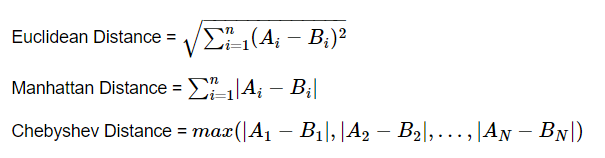As with most mathematical concepts, distance metrics are often easier to understand with a concrete example to visualize. Consider Point A = (0,0) and Point B = (3,4) in 2D feature space.

``````sns.set_style('darkgrid')
fig = plt.figure()
axes.scatter(x=[0,3],y=[0,4],s = 50)
axes.plot([0,3],[0,4],c='blue')
axes.annotate('X',[1.5,1.8],fontsize = 14,fontweight = 'bold')
axes.plot([0,0],[0,4],c='blue')
axes.annotate('Y',[-0.1,1.8],fontsize = 14,fontweight = 'bold')
axes.plot([0,3],[4,4],c='blue')
axes.annotate('Z',[1.5,4.1],fontsize = 14,fontweight = 'bold')
axes.annotate('(0,0)',[0.1,0.0],fontsize = 14,fontweight = 'bold')
axes.annotate('(3,4)',[2.85,3.7],fontsize = 14,fontweight = 'bold')
axes.grid(lw=1)
plt.show()
``````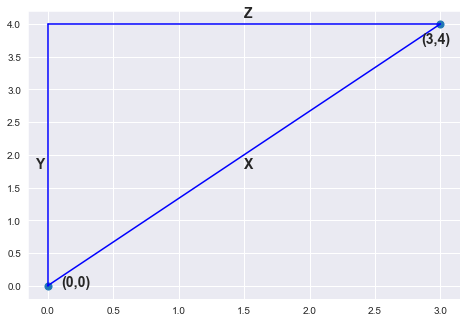#python #machine-learning #siri #k-nearest-neighbors #crossvalidation